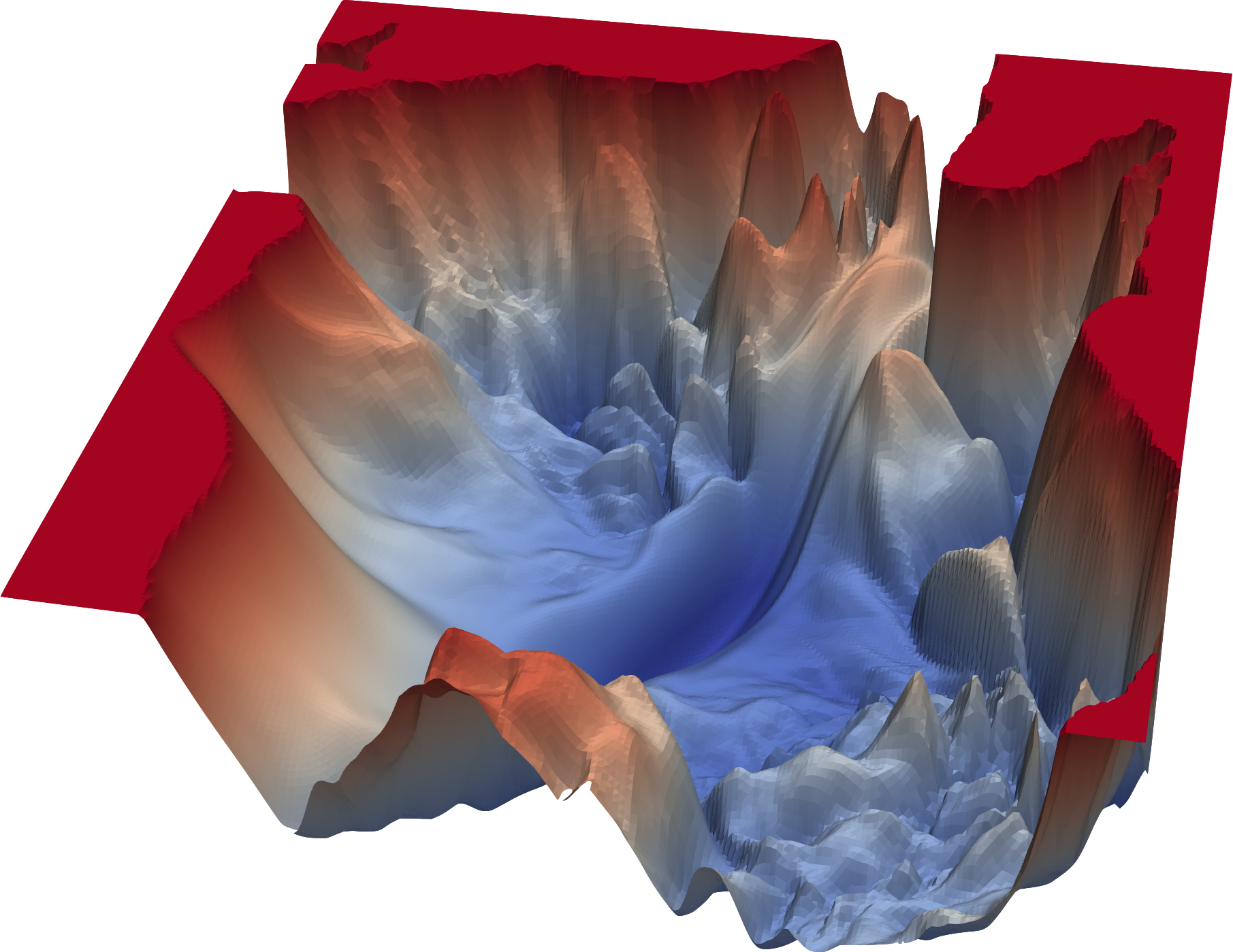# Chapter 01: Mathematical Concepts

This chapter introduces the basic mathematical concepts that are prerequisite for most optimization methods.

• Chapter 1.1: Differentiability

In this section, we refresh the basic concept of differentiability for univariate and multivariate functions and introduce concepts such as the Jacobi- or Hessian Matrix.

• Chapter 1.2: Taylor Approximation

In this section, we explain both the univariate and the multivariate Taylor polynomial. Download &raquo;slides-concepts-2-taylor.pdf&laquo;

• Chapter 1.3: Convexity

In this section, we introduce the concept of convexity and briefly touch upon its importance for optimization.

• Chapter 1.4: Conditions for optimality